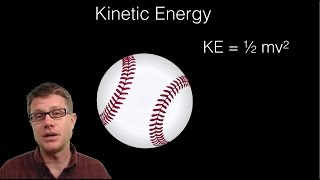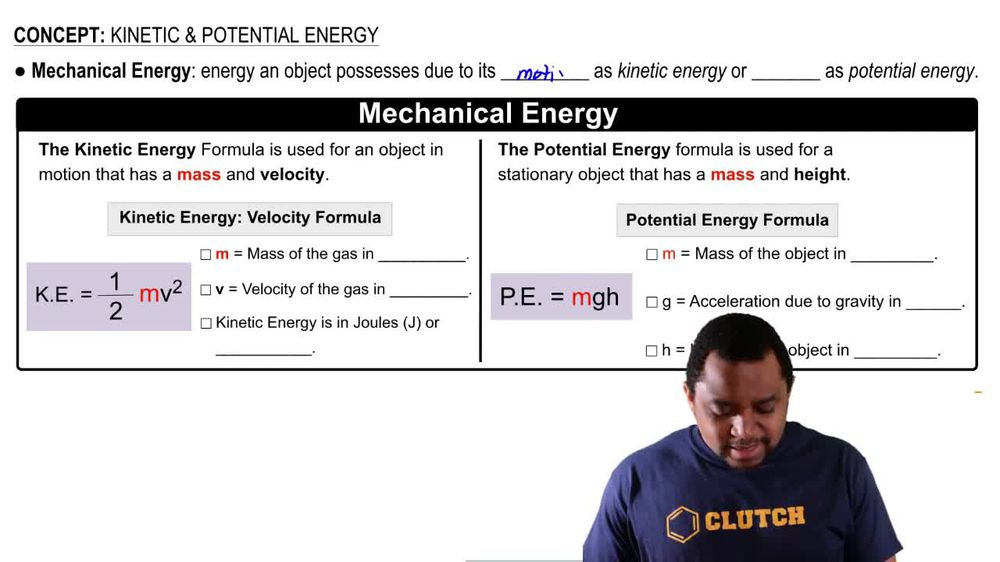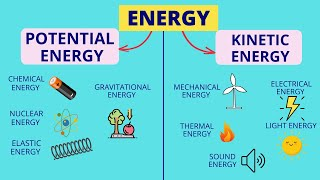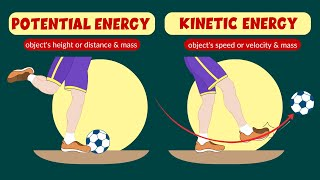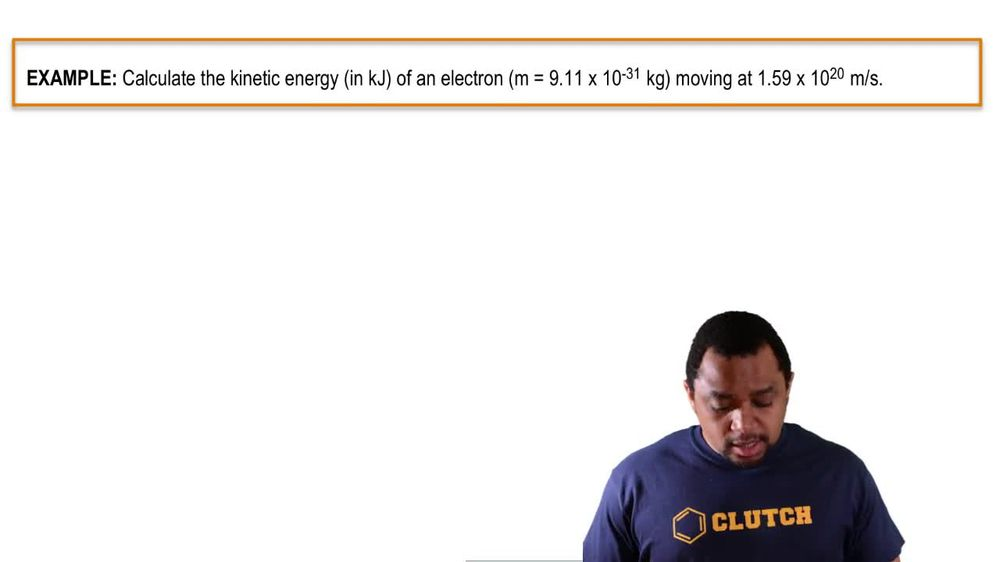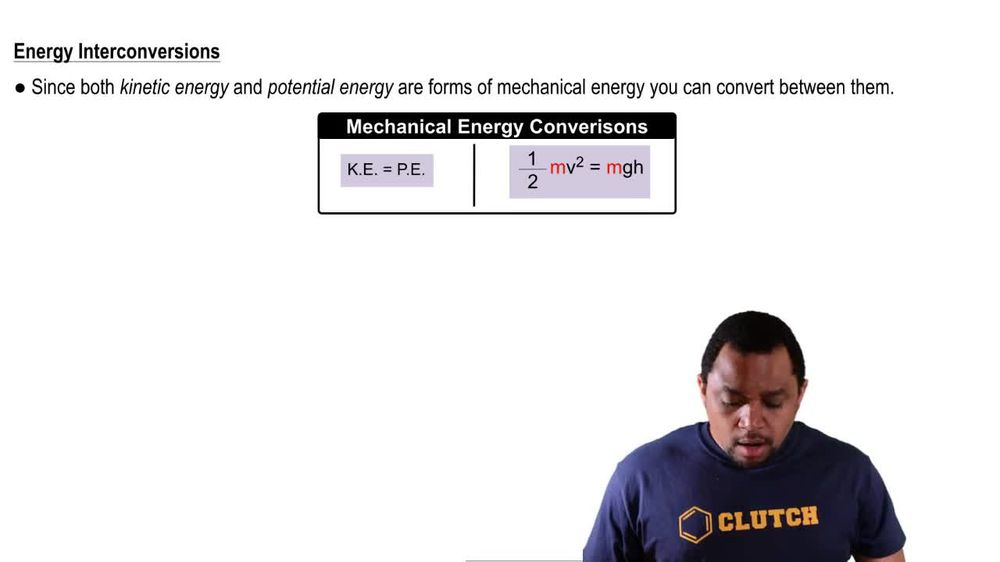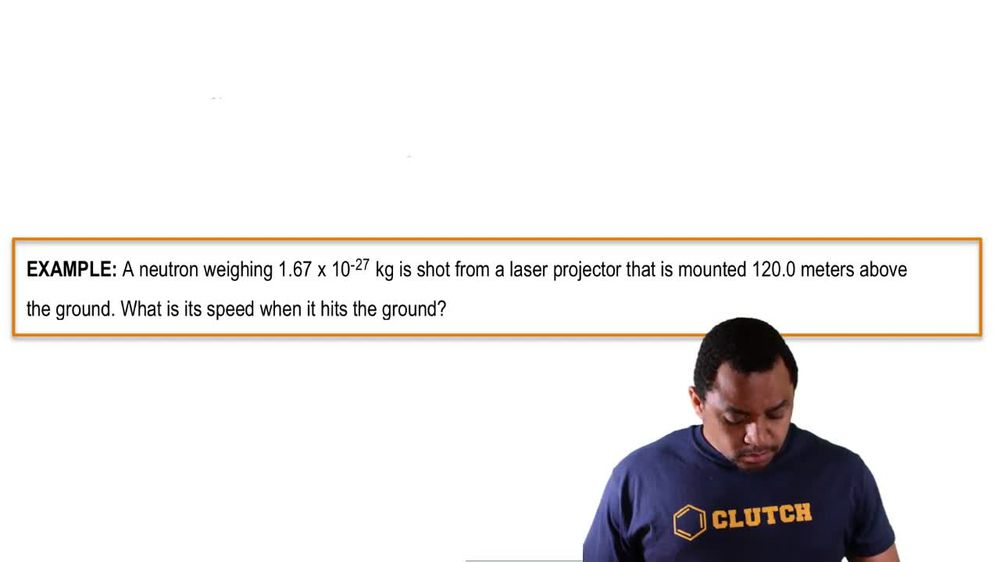Start typing, then use the up and down arrows to select an option from the list.
1. 8. Thermochemistry2. Kinetic & Potential Energy# Kinetic & Potential Energy

by Jules Bruno
369 views
1
0
kinetic energy and potential energy are connected to one another because they form part off mechanical energy. Now mechanical energy is energy and object possesses, due to its motion as kinetic energy or its position as potential energy. Now, with Connecticut and energy and potential energy, we have formulas that are important to remember. So the kinetic energy formula is used for an object in motion that has a mass and velocity which is connected to its speed. Here we say kinetic energy, which is abbreviated as K e, equals half times m times v squared here am equals the mass of the of the gas in kilograms and V equals velocity of the gas in meters per second. Kinetic energy ke e is in jewels, which is capital J, or just think about it. If this is in kilograms and were squaring meters per second, that would mean that jewels are equivalent to kilograms. Times meter squared over second squared potential energy formula is used for a stationary object that has a mass and ah, height. Now here we say, potential energy, which is abbreviated as p e equals m times g times H here, M equals the mass of the object again in kilograms. And here we're gonna say G is acceleration due to gravity. And here on earth, that is 9.8 meters over seconds squared. So that's gonna be as close as we get to physics this semester. So just keep that in mind in terms of acceleration due to gravity. For those of you are gonna take physics or have taken physics, you should know this type of idea and then h equals the height of the object in meters. So just remember, kinetic energy and potential energy are different, but they formed the sum of mechanical energy.